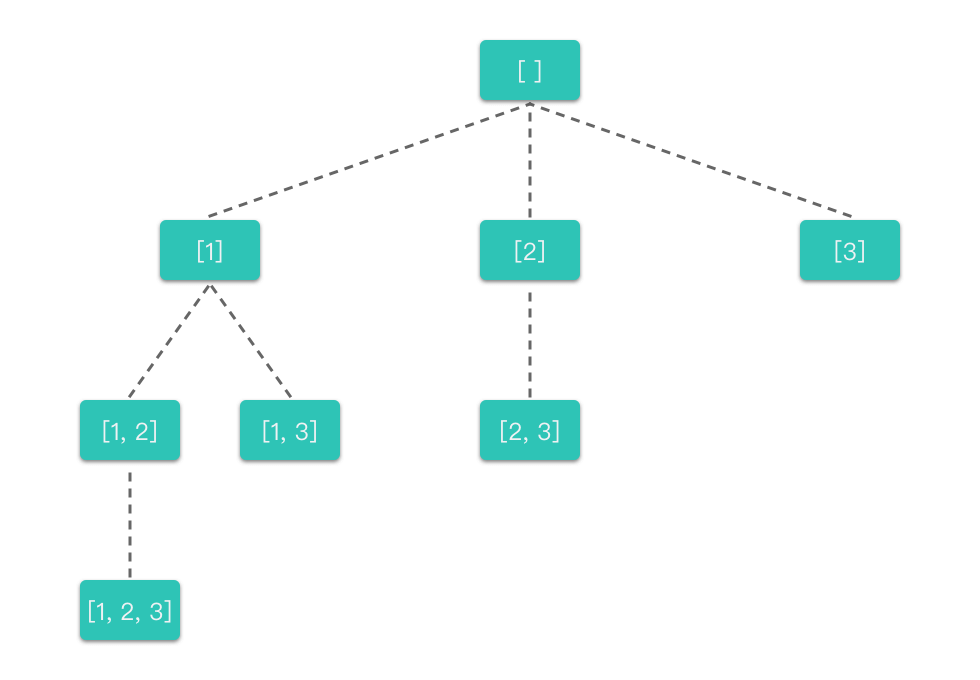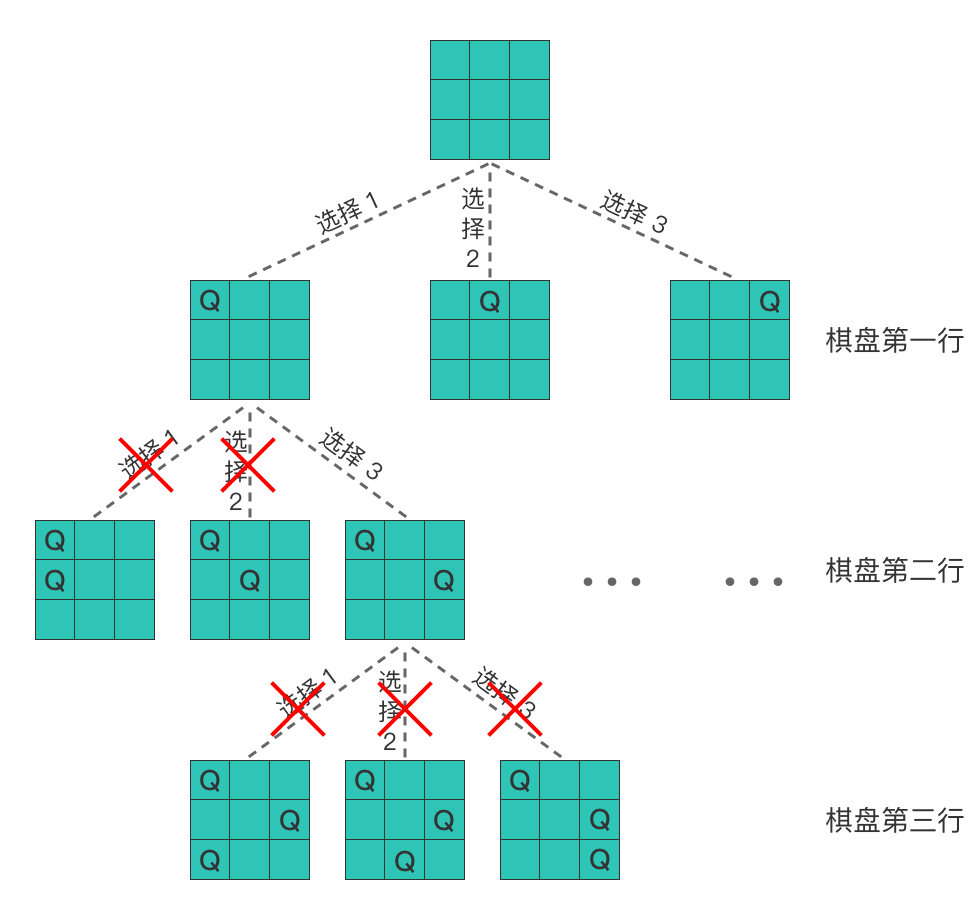# # 回溯算法知识

## # 1. 回溯算法简介

1. 找到一个可能存在的正确答案；
2. 在尝试了所有可能的分布方法之后宣布该问题没有答案。

## # 2. 从全排列问题开始理解回溯算法

1. 选择以 1 为开头的全排列。
1. 选择以 2 为中间数字的全排列，则最后数字只能选择 3。即排列为：[1, 2, 3]
2. 撤销选择以 3 为最后数字的全排列，再撤销选择以 2 为中间数字的全排列。然后选择以 3 为中间数字的全排列，则最后数字只能选择 2，即排列为：[1, 3, 2]
2. 撤销选择以 2 为最后数字的全排列，再撤销选择以 3 为中间数字的全排列，再撤销选择以 1 为开头的全排列。然后选择以 2 开头的全排列。
1. 选择以 1 为中间数字的全排列，则最后数字只能选择 3。即排列为：[2, 1, 3]
2. 撤销选择以 3 为最后数字的全排列，再撤销选择以 1 为中间数字的全排列。然后选择以 3 为中间数字的全排列，则最后数字只能选择 1，即排列为：[2, 3, 1]
3. 撤销选择以 1 为最后数字的全排列，再撤销选择以 3 为中间数字的全排列，再撤销选择以 2 为开头的全排列，选择以 3 开头的全排列。
1. 选择以 1 为中间数字的全排列，则最后数字只能选择 2。即排列为：[3, 1, 2]
2. 撤销选择以 2 为最后数字的全排列，再撤销选择以 1 为中间数字的全排列。然后选择以 2 为中间数字的全排列，则最后数字只能选择 1，即排列为：[3, 2, 1]

• 按顺序枚举每一位上可能出现的数字，之前已经出现的数字在接下来要选择的数字中不能再次出现。
• 对于每一位，进行如下几步：
1. 选择元素：从可选元素列表中选择一个之前没有出现过的元素。
2. 递归搜索：从选择的元素出发，一层层地递归搜索剩下位数，直到遇到边界条件时，不再向下搜索。
3. 撤销选择：一层层地撤销之前选择的元素，转而进行另一个分支的搜索。直到完全遍历完所有可能的路径。

• 每一层中有一个或多个不同的节点，这些节点以及节点所连接的分支代表了「不同的选择」。
• 每一个节点代表了求解全排列问题的一个「状态」，这些状态是通过「不同的值」来表现的。
• 每向下递推一层就是在「可选元素列表」中选择一个「元素」加入到「当前状态」。
• 当一个决策分支探索完成之后，会逐层向上进行回溯。
• 每向上回溯一层，就是把所选择的「元素」从「当前状态」中移除，回退到没有选择该元素时的状态（或者说重置状态），从而进行其他分支的探索。

class Solution:
def permute(self, nums: List[int]) -> List[List[int]]:
res = []    # 存放所有符合条件结果的集合
path = []   # 存放当前符合条件的结果
def backtracking(nums):             # nums 为选择元素列表
if len(path) == len(nums):      # 说明找到了一组符合条件的结果
res.append(path[:])         # 将当前符合条件的结果放入集合中
return

for i in range(len(nums)):      # 枚举可选元素列表
if nums[i] not in path:     # 从当前路径中没有出现的数字中选择
path.append(nums[i])    # 选择元素
backtracking(nums)      # 递归搜索
path.pop()              # 撤销选择

backtracking(nums)
return res


## # 3. 回溯算法的通用模板

res = []    # 存放所欲符合条件结果的集合
path = []   # 存放当前符合条件的结果
def backtracking(nums):             # nums 为选择元素列表
if 遇到边界条件:                  # 说明找到了一组符合条件的结果
res.append(path[:])         # 将当前符合条件的结果放入集合中
return

for i in range(len(nums)):      # 枚举可选元素列表
path.append(nums[i])        # 选择元素
backtracking(nums)          # 递归搜索
path.pop()                  # 撤销选择

backtracking(nums)


## # 4. 回溯算法三步走

1. 根据所给问题，定义问题的解空间：要定义合适的解空间，包括解的组织形式和显约束。
• 解的组织形式：将解的组织形式都规范为⼀个 n 元组 ${x_1, x_2 …, x_n}$
• 显约束：对解分量的取值范围的限定，可以控制解空间的大小。
2. 确定解空间的组织结构：解空间的组织结构通常以解空间树的方式形象地表达，根据解空间树的不同，解空间分为⼦集树、排列树、m 叉树等。
3. 搜索解空间：按照深度优先搜索策略，根据隐约束（约束函数和限界函数），在解空间中搜索问题的可⾏解或最优解。当发现当 前节点不满⾜求解条件时，就回溯，尝试其他路径。
• 如果问题只是求可⾏解，则只需设定约束函数即可，如果要求最优解，则需要设定约束函数和限界函数。

1. 明确所有选择：画出搜索过程的决策树，根据决策树来确定搜索路径。
2. 明确终止条件：推敲出递归的终止条件，以及递归终止时的要执行的处理方法。
3. 将决策树和终止条件翻译成代码：
1. 定义回溯函数（明确函数意义、传入参数、返回结果等）。
2. 书写回溯函数主体（给出约束条件、选择元素、递归搜索、撤销选择部分）。
3. 明确递归终止条件（给出递归终止条件，以及递归终止时的处理方法）。

### # 4.3 将决策树和终止条件翻译成代码

1. 定义回溯函数（明确函数意义、传入参数、返回结果等）。
2. 书写回溯函数主体（给出约束条件、选择元素、递归搜索、撤销选择部分）。
3. 明确递归终止条件（给出递归终止条件，以及递归终止时的处理方法）。

#### # 4.3.1 定义回溯函数

• 传入参数和全局变量：是由递归搜索阶段时的「当前状态」来决定的。最好是能通过传入参数和全局变量直接记录「当前状态」。

• 返回结果：返回结果是在遇到递归终止条件时，需要向上一层函数返回的信息。

#### # 4.3.2 书写回溯函数主体

for i in range(len(nums)):          # 枚举可选元素列表
if 满足约束条件:                  # 约束条件
path.append(nums[i])        # 选择元素
backtracking(nums)          # 递归搜索
path.pop()                  # 撤销选择


## # 5. 回溯算法的应用

### # 5.1 子集

#### # 5.1.2 题目大意

• $1 \le nums.length \le 10$
• $-10 \le nums[i] \le 10$
• nums 中的所有元素互不相同。

• 示例 1：
输入 nums = [1,2,3]


• 示例 2：
输入：nums = 



#### # 5.1.3 解题思路

##### # 思路 1：回溯算法

1. 明确所有选择：根据数组中每个位置上的元素选与不选两种选择，画出决策树，如下图所示。
•1. 明确终止条件

• 当遍历到决策树的叶子节点时，就终止了。即当前路径搜索到末尾时，递归终止。
2. 将决策树和终止条件翻译成代码：

1. 定义回溯函数：

• backtracking(nums, index): 函数的传入参数是 nums（可选数组列表）和 index（代表当前正在考虑元素是 nums[i] ），全局变量是 res（存放所有符合条件结果的集合数组）和 path（存放当前符合条件的结果）。
• backtracking(nums, index): 函数代表的含义是：在选择 nums[index] 的情况下，递归选择剩下的元素。
2. 书写回溯函数主体（给出选择元素、递归搜索、撤销选择部分）。

• 从当前正在考虑元素，到数组结束为止，枚举出所有可选的元素。对于每一个可选元素：
• 约束条件：之前选过的元素不再重复选用。每次从 index 位置开始遍历而不是从 0 位置开始遍历就是为了避免重复。集合跟全排列不一样，子集中 {1, 2}{2, 1} 是等价的。为了避免重复，我们之前考虑过的元素，就不再重复考虑了。
• 选择元素：将其添加到当前子集数组 path 中。
• 递归搜索：在选择该元素的情况下，继续递归考虑下一个位置上的元素。
• 撤销选择：将该元素从当前子集数组 path 中移除。
for i in range(index, len(nums)):   # 枚举可选元素列表
path.append(nums[i])            # 选择元素
backtracking(nums, i + 1)       # 递归搜索
path.pop()                      # 撤销选择

1. 明确递归终止条件（给出递归终止条件，以及递归终止时的处理方法）。
• 当遍历到决策树的叶子节点时，就终止了。也就是当正在考虑的元素位置到达数组末尾（即 start >= len(nums)）时，递归停止。
• 从决策树中也可以看出，子集需要存储的答案集合应该包含决策树上所有的节点，应该需要保存递归搜索的所有状态。所以无论是否达到终止条件，我们都应该将当前符合条件的结果放入到集合中。
##### # 思路 1：代码
class Solution:
def subsets(self, nums: List[int]) -> List[List[int]]:
res = []  # 存放所有符合条件结果的集合
path = []  # 存放当前符合条件的结果
def backtracking(nums, index):          # 正在考虑可选元素列表中第 index 个元素
res.append(path[:])                 # 将当前符合条件的结果放入集合中
if index >= len(nums):              # 遇到终止条件（本题）
return

for i in range(index, len(nums)):   # 枚举可选元素列表
path.append(nums[i])            # 选择元素
backtracking(nums, i + 1)       # 递归搜索
path.pop()                      # 撤销选择

backtracking(nums, 0)
return res

##### # 思路 1：复杂度分析
• 时间复杂度$O(n \times 2^n)$，其中 $n$ 指的是数组 nums 的元素个数，$2^n$ 指的是所有状态数。每种状态需要 $O(n)$ 的时间来构造子集。
• 空间复杂度$O(n)$，每种状态下构造子集需要使用 $O(n)$ 的空间。

### # 5.2 N 皇后

#### # 5.2.2 题目大意

• n 皇后问题：将 n 个皇后放置在 n * n 的棋盘上，并且使得皇后彼此之间不能攻击。
• 皇后彼此不能相互攻击：指的是任何两个皇后都不能处于同一条横线、纵线或者斜线上。
• $1 \le n \le 9$

• 示例 1：
输入：n = 4



#### # 5.2.3 解题思路

##### # 思路 1：回溯算法

1. 明确所有选择：根据棋盘中当前行的所有列位置上是否选择放置皇后，画出决策树，如下图所示。

•2. 明确终止条件

• 当遍历到决策树的叶子节点时，就终止了。也就是在最后一行放置完皇后时，递归终止。
3. 将决策树和终止条件翻译成代码：

1. 定义回溯函数：

• 首先我们先使用一个 n * n 大小的二维矩阵 chessboard 来表示当前棋盘，chessboard 中的字符 Q 代表皇后，. 代表空位，初始都为 .
• 然后定义回溯函数 backtrack(chessboard, row):  函数的传入参数是 chessboard（棋盘数组）和 row（代表当前正在考虑放置第 row 行皇后），全局变量是 res（存放所有符合条件结果的集合数组）。
• backtrack(chessboard, row): 函数代表的含义是：在放置好第 row 行皇后的情况下，递归放置剩下行的皇后。
2. 书写回溯函数主体（给出选择元素、递归搜索、撤销选择部分）。

• 枚举出当前行所有的列。对于每一列位置：
• 约束条件：定义一个判断方法，先判断一下当前位置是否与之前棋盘上放置的皇后发生冲突，如果不发生冲突则继续放置，否则则继续向后遍历判断。
• 选择元素：选择 row, col 位置放置皇后，将其棋盘对应位置设置为 Q
• 递归搜索：在该位置放置皇后的情况下，继续递归考虑下一行。
• 撤销选择：将棋盘上 row, col 位置设置为 .
# 判断当前位置 row, col 是否与之前放置的皇后发生冲突
def isValid(self, n: int, row: int, col: int, chessboard: List[List[str]]):
for i in range(row):
if chessboard[i][col] == 'Q':
return False

i, j = row - 1, col - 1
while i >= 0 and j >= 0:
if chessboard[i][j] == 'Q':
return False
i -= 1
j -= 1
i, j = row - 1, col + 1
while i >= 0 and j < n:
if chessboard[i][j] == 'Q':
return False
i -= 1
j += 1

return True

for col in range(n):                            # 枚举可放置皇后的列
if self.isValid(n, row, col, chessboard):   # 如果该位置与之前放置的皇后不发生冲突
chessboard[row][col] = 'Q'              # 选择 row, col 位置放置皇后
backtrack(row + 1, chessboard)          # 递归放置 row + 1 行之后的皇后
chessboard[row][col] = '.'              # 撤销选择 row, col 位置

1. 明确递归终止条件（给出递归终止条件，以及递归终止时的处理方法）。
• 当遍历到决策树的叶子节点时，就终止了。也就是在最后一行放置完皇后（即 row == n）时，递归停止。
• 递归停止时，将当前符合条件的棋盘转换为答案需要的形式，然后将其存入答案数组 res 中即可。
##### # 思路 1：代码
class Solution:
res = []
def backtrack(self, n: int, row: int, chessboard: List[List[str]]):
if row == n:
temp_res = []
for temp in chessboard:
temp_str = ''.join(temp)
temp_res.append(temp_str)
self.res.append(temp_res)
return
for col in range(n):
if self.isValid(n, row, col, chessboard):
chessboard[row][col] = 'Q'
self.backtrack(n, row + 1, chessboard)
chessboard[row][col] = '.'

def isValid(self, n: int, row: int, col: int, chessboard: List[List[str]]):
for i in range(row):
if chessboard[i][col] == 'Q':
return False

i, j = row - 1, col - 1
while i >= 0 and j >= 0:
if chessboard[i][j] == 'Q':
return False
i -= 1
j -= 1
i, j = row - 1, col + 1
while i >= 0 and j < n:
if chessboard[i][j] == 'Q':
return False
i -= 1
j += 1

return True

def solveNQueens(self, n: int) -> List[List[str]]:
self.res.clear()
chessboard = [['.' for _ in range(n)] for _ in range(n)]
self.backtrack(n, 0, chessboard)
return self.res

##### # 思路 1：复杂度分析
• 时间复杂度$O(n!)$，其中 $n$ 是皇后数量。
• 空间复杂度$O(n^2)$，其中 $n$ 是皇后数量。递归调用层数不会超过 $n$，每个棋盘的空间复杂度为 $O(n^2)$，所以空间复杂度为 $O(n^2)$